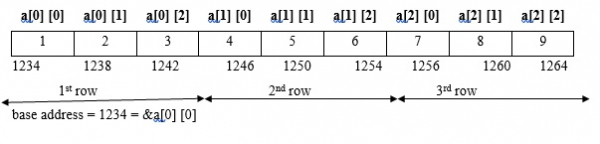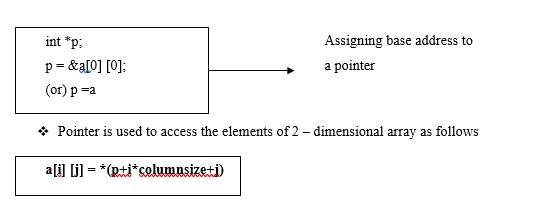# Explain pointers and two-dimensional array in C language

Pointer is a variable that stores the address of another variable.

## Features

• Pointer saves the memory space.

• Execution time of pointer is faster because of direct access to memory location.

• With the help of pointers, the memory is accessed efficiently, i.e., memory is allocated and deallocated dynamically.

• Pointers are used with data structures.

## Pointers and two dimensional arrays

Memory allocation for a two-dimensional array is as follows −

int a  = {1,2,3,4,5,6,7,8,9};a  = *(1234 + 1*3+2)
= *(1234 + 3+2)
= *(1234 + 5*4) // 4 is Scale factor
= * (1234+20)
= *(1254)
a  = 6

## Example

Following is the C program for pointers and two-dimensional array −

#include<stdio.h>
main ( ){
int a , i,j;
int *p;
clrscr ( );
printf ("Enter elements of 2D array");
for (i=0; i<3; i++){
for (j=0; j<3; j++){
scanf ("%d", &a[i] [j]);
}
}
p = &a ;
printf ("elements of 2d array are");
for (i=0; i<3; i++){
for (j=0; j<3; j++){
printf ("%d \t", *(p+i*3+j));
}
printf ("");
}
getch ( );
}

## Output

When the above program is executed, it produces the following result −

enter elements of 2D array
1 2 3 4 5 6 7 8 9
Elements of 2D array are
1 2 3
4 5 6
7 8 9

Updated on: 17-Mar-2021

4K+ Views